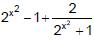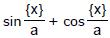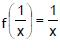# Test: Maxima And Minima (Competition Level) - 2

## 19 Questions MCQ Test Mathematics (Maths) Class 12 | Test: Maxima And Minima (Competition Level) - 2

Description
This mock test of Test: Maxima And Minima (Competition Level) - 2 for JEE helps you for every JEE entrance exam. This contains 19 Multiple Choice Questions for JEE Test: Maxima And Minima (Competition Level) - 2 (mcq) to study with solutions a complete question bank. The solved questions answers in this Test: Maxima And Minima (Competition Level) - 2 quiz give you a good mix of easy questions and tough questions. JEE students definitely take this Test: Maxima And Minima (Competition Level) - 2 exercise for a better result in the exam. You can find other Test: Maxima And Minima (Competition Level) - 2 extra questions, long questions & short questions for JEE on EduRev as well by searching above.
QUESTION: 1

Solution:
QUESTION: 2

Solution:
QUESTION: 3

### Least value of the function, f(x)=is

Solution:

(2x2 - 1) + 2/(2x2 + 1)
AM ≥ GM
f(x) = (2x2 + 1) + 2/(2x2 + 1) - 2
[(2x2 - 1) + 2/(2x2 + 1)]/2
⇒ [(2x2 - 1) * 2/(2x2 + 1)]^½
⇒ [(2x2 - 1) * 2/(2x2 + 1)]^½ ≥ 2(2)^½ - 2
⇒ f(x) ≥ 2(2)^½ - 2

QUESTION: 4

A triangular park is enclosed on two sides by a fence and on the third side by a straight river bank. The two sides having fence are of same length x. The maximum area enclosed by the park is

Solution:
QUESTION: 5

The co-ordinate of the point for minimum value of z = 7x – 8y subject to the conditions x + y – 20 £ 0, y ³ 5, x ³ 0, y ³ 0

Solution:
QUESTION: 6

The equation x3 – 3x + [a] = 0, will have three real and distinct roots if

(where [*] denotes the greatest integer function)

Solution:
QUESTION: 7

Let f(x) =. Then the set of values of a for which f can attain its maximum values is

(where a>0 and { * } denotes the fractional part function)

Solution:
QUESTION: 8

A function is defined as f(x) = ax2 – b|x| where a and b are constants then at x = 0 we will have a maxima of f(x) if

Solution:
QUESTION: 9

A and B are the points (2, 0) and (0, 2) respectively. The coordinates of the point P on the line 2x+3y+1=0 are

Solution:
QUESTION: 10

The maximum value of f(x) = 2bx2 – x4 – 3b is g(b), where b > 0, if b varies then the minimum value of g(b) is

Solution:
QUESTION: 11

Number of solution(s) satisfying the equation, 3x2 – 2x3 = log2 (x2 + 1) – log2 x is

Solution:
QUESTION: 12

If a2x4 + b2 y4 = c6, then the maximum value of xy is

Solution:
QUESTION: 13

Maximum and minimum value of f(x) = max (sin t), 0 < t < x, 0 £ x £ 2p are

Solution:
QUESTION: 14

The function `f' is defined by f(x) = xp (1 – x)q for all x Î R, where p, q are positive integers, has a maximum value, for x equal to

Solution:
QUESTION: 15

The maximum slope of the curve y=–x3+3x2+2x–27 will be

Solution:
QUESTION: 16

Two points A(1, 4) & B(3, 0) are given on the ellipse 2x2 + y2 = 18. The co-ordinates of a point C on the ellipse such that the area of the triangle ABC is greatest is

Solution:
QUESTION: 17

The lateral edge of a regular hexagonal pyramid is 1 cm. If the volume is maximum, then its height must be equal to

Solution:
QUESTION: 18

Let f(x) = 5x – 2x2 + 2; x ∈ N then the maximum value of f(x) is

Solution:
QUESTION: 19

The maximum value of f(x), if f(x) +, x ∈ domain of f

Solution: